Comparing rational numbers

Chapter 9 Class 7 Rational Numbers
Concept wise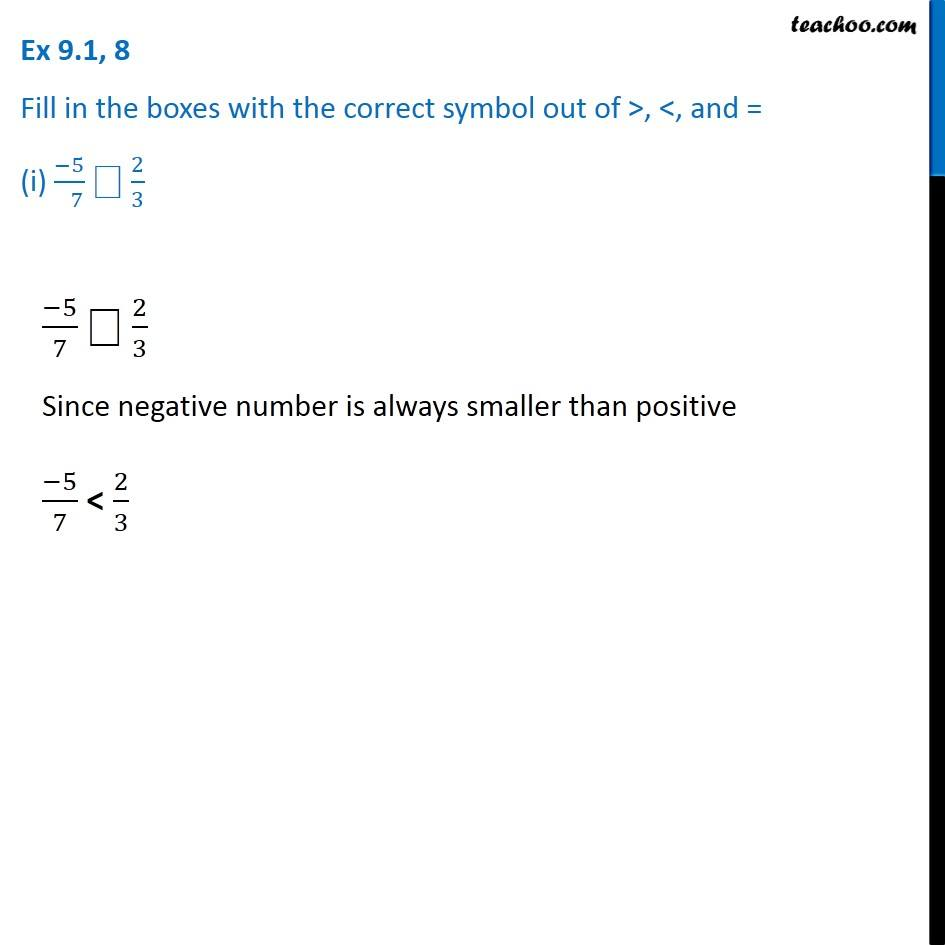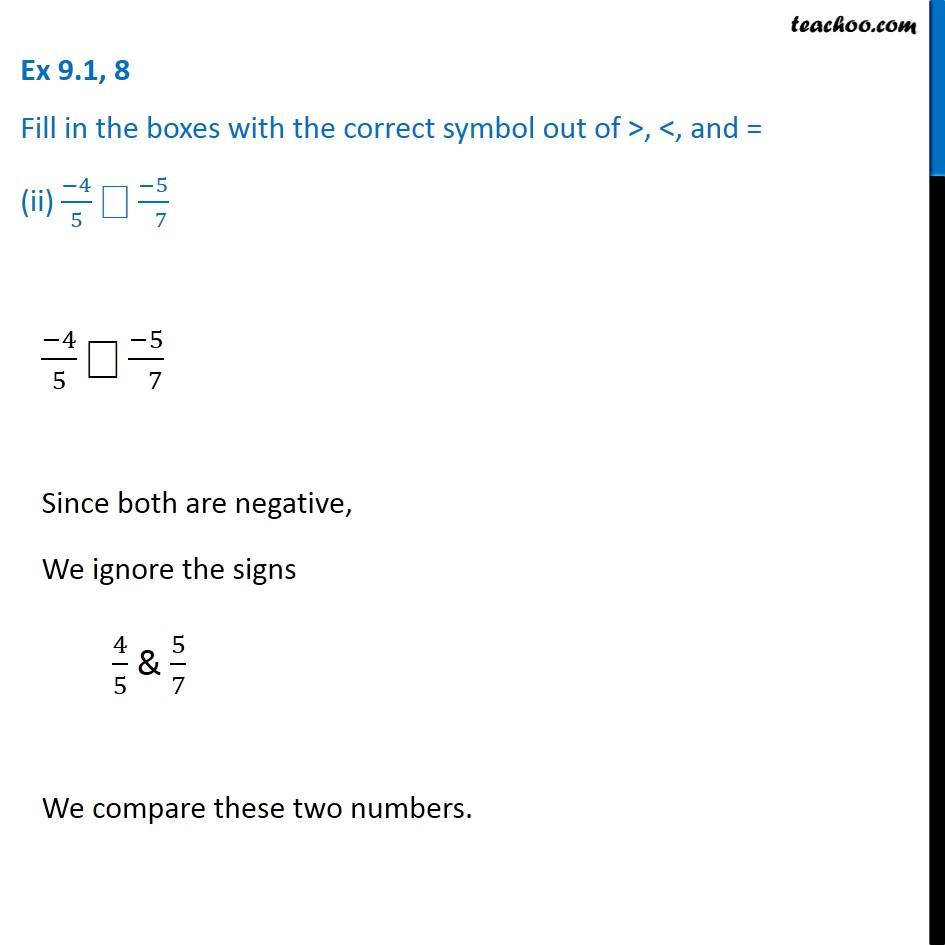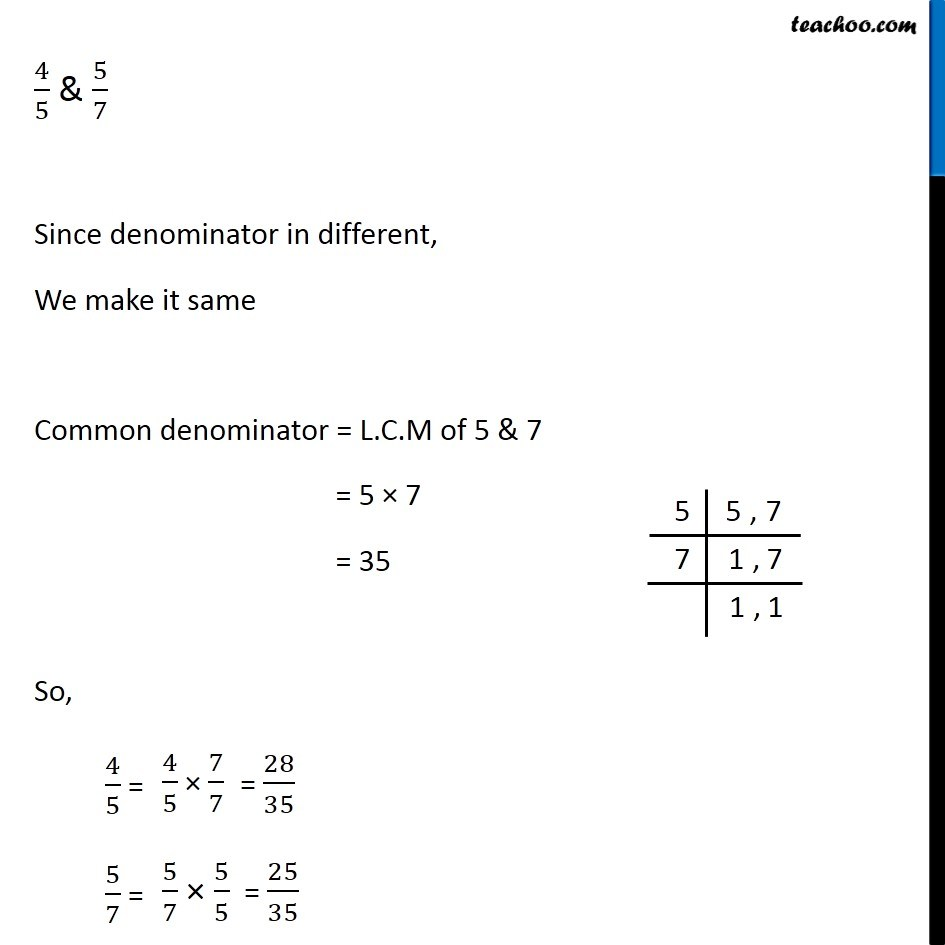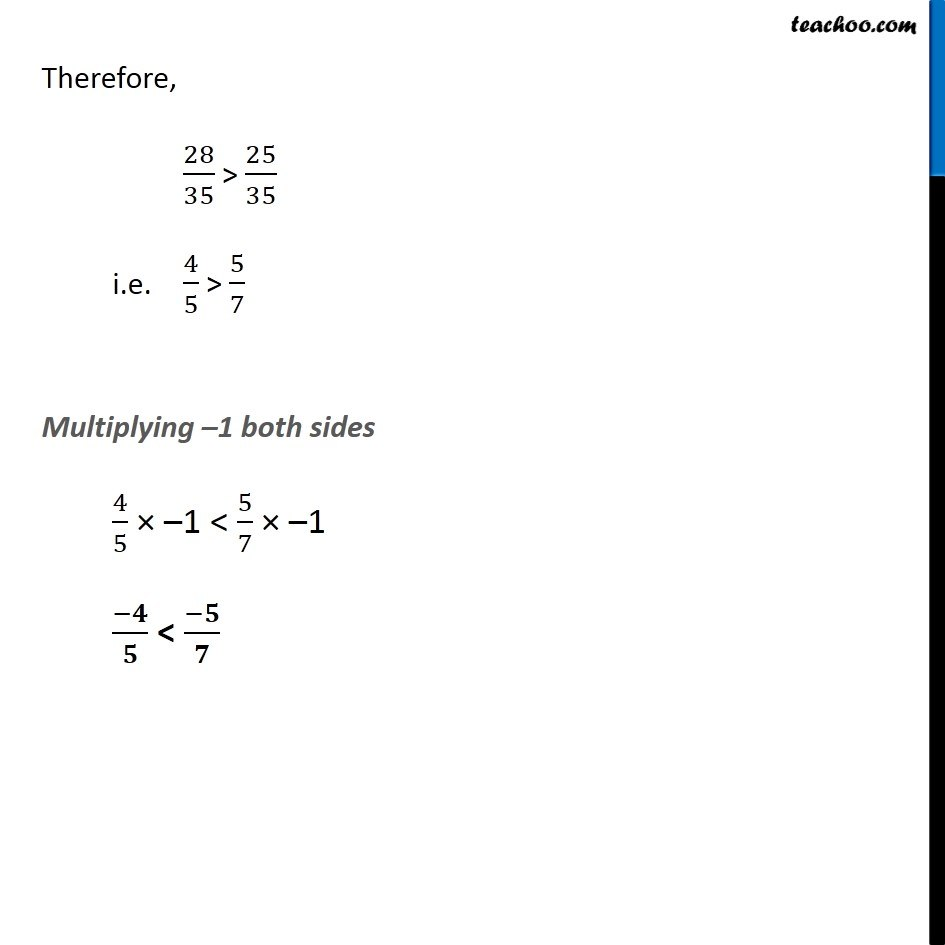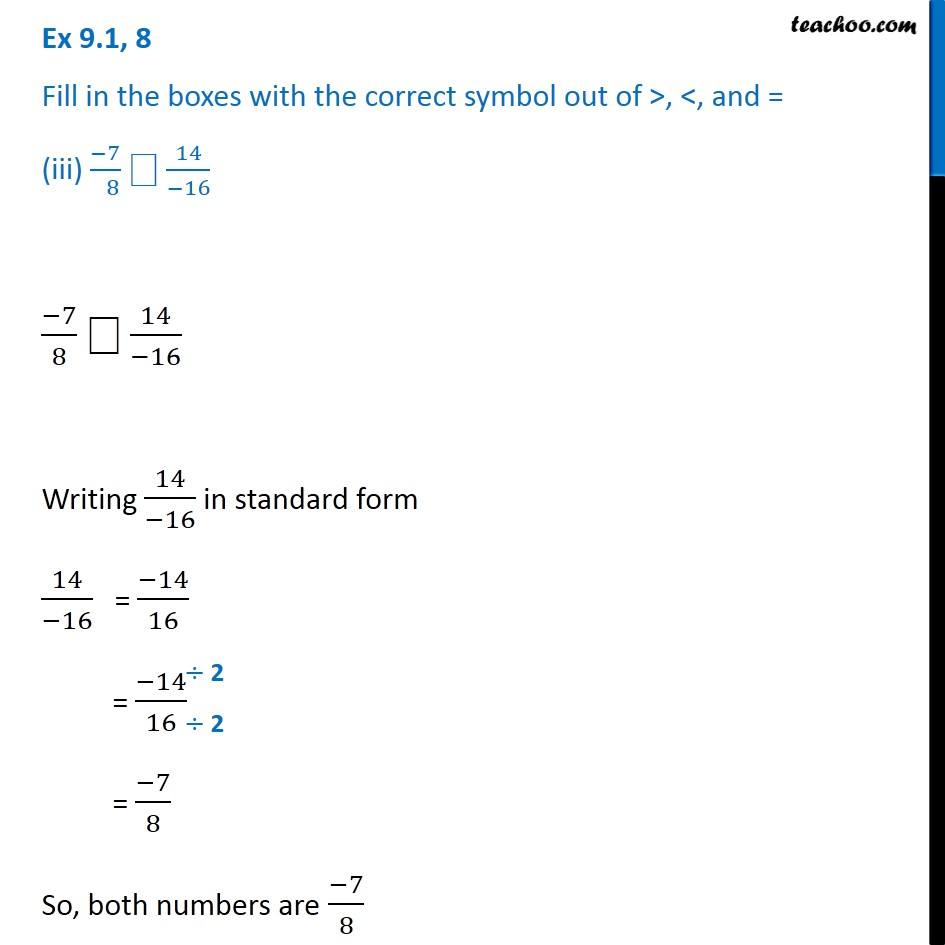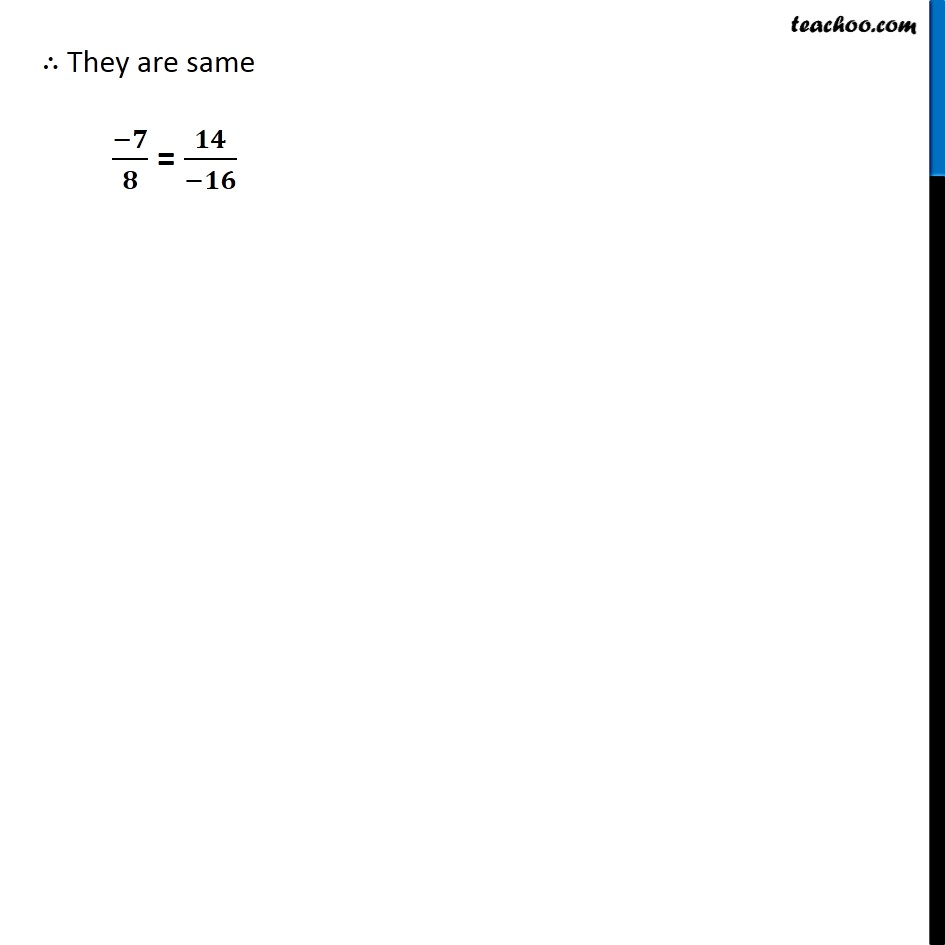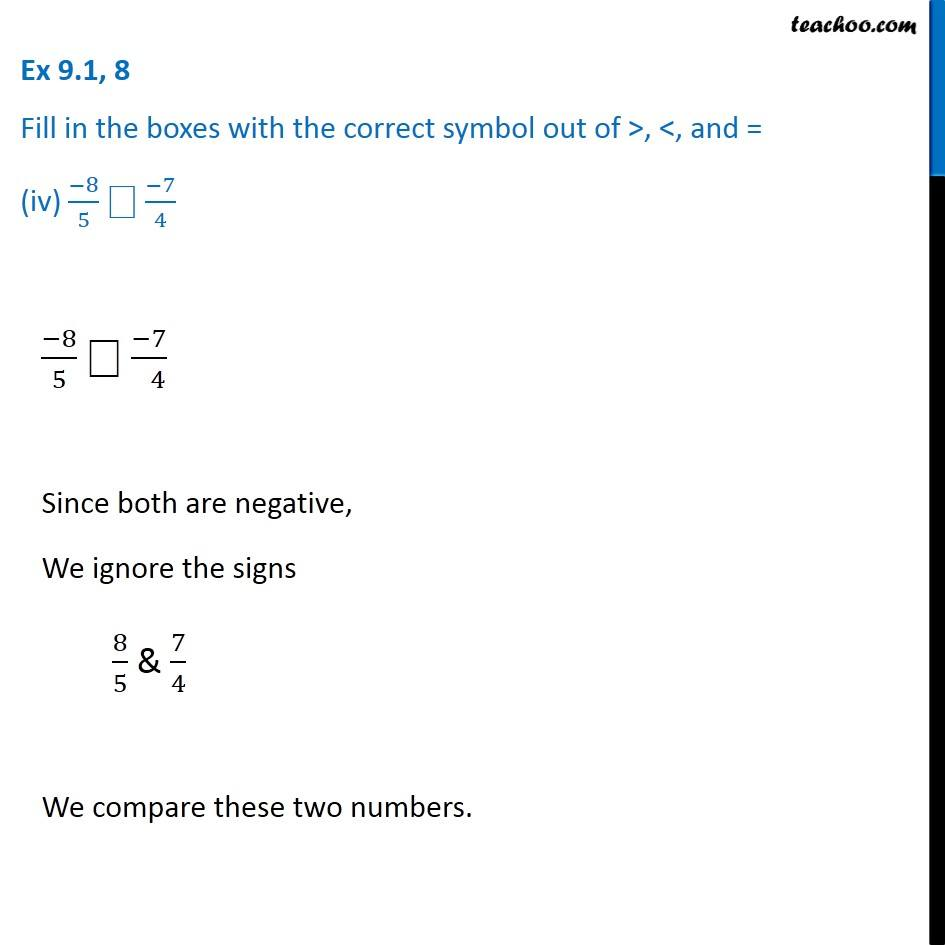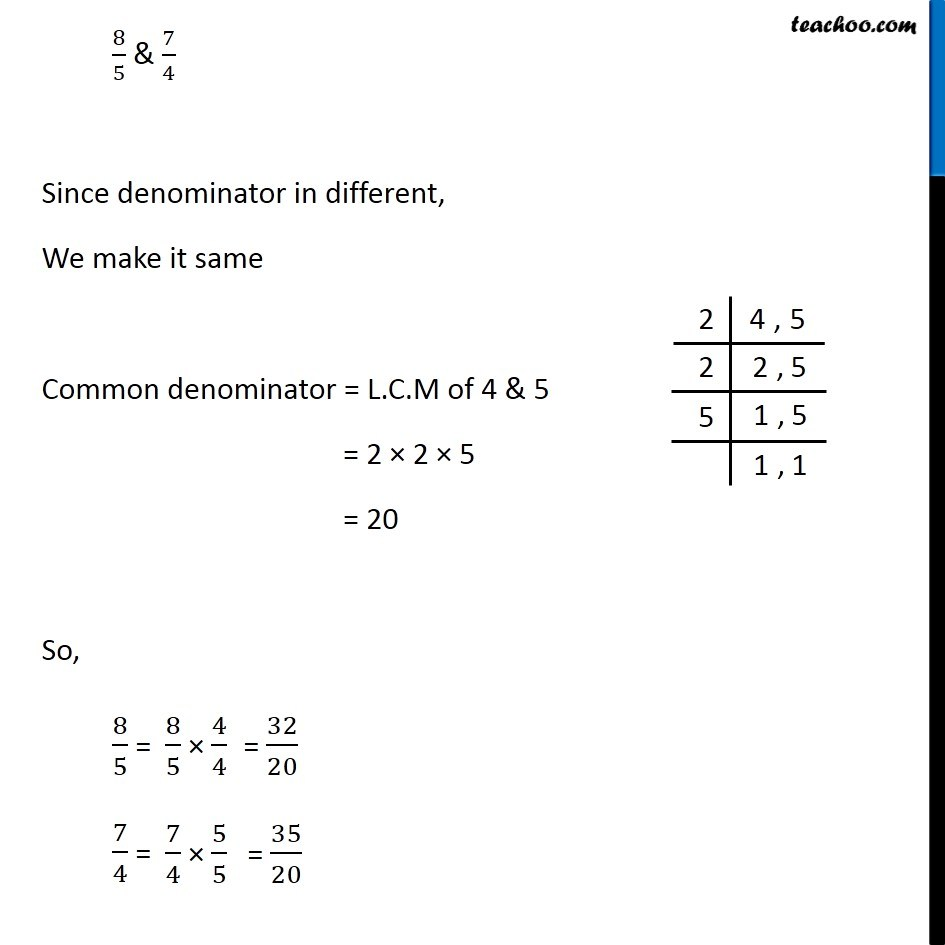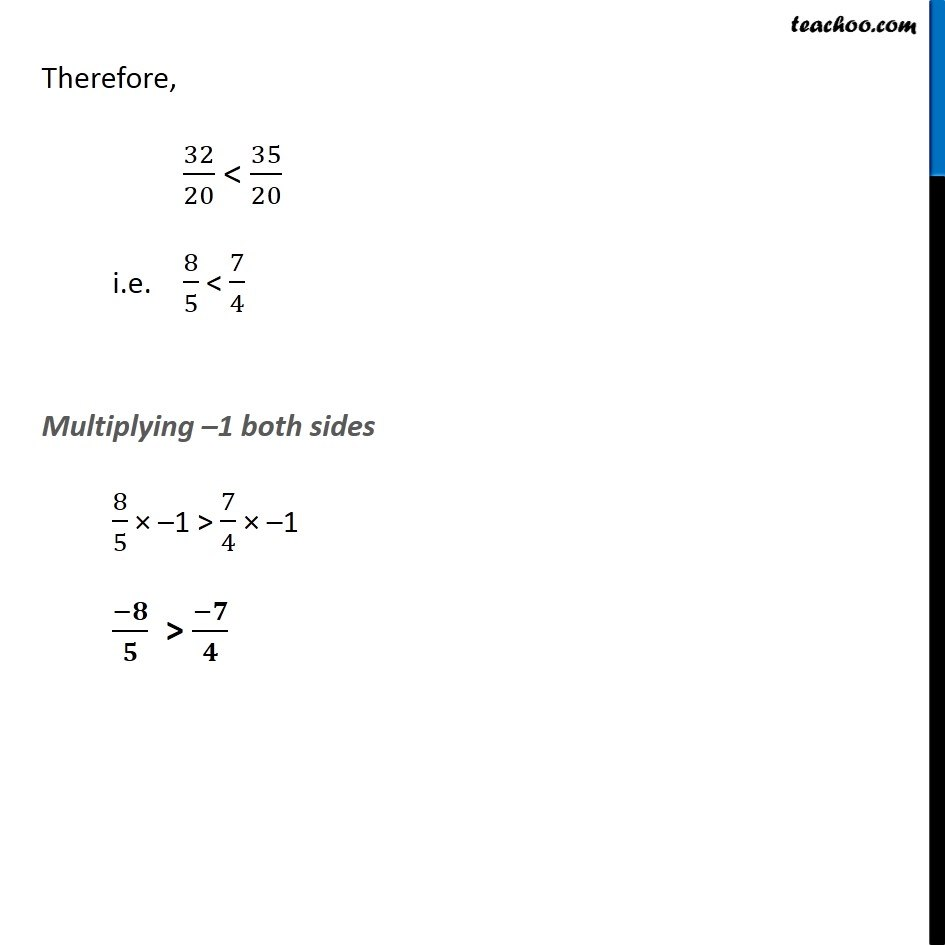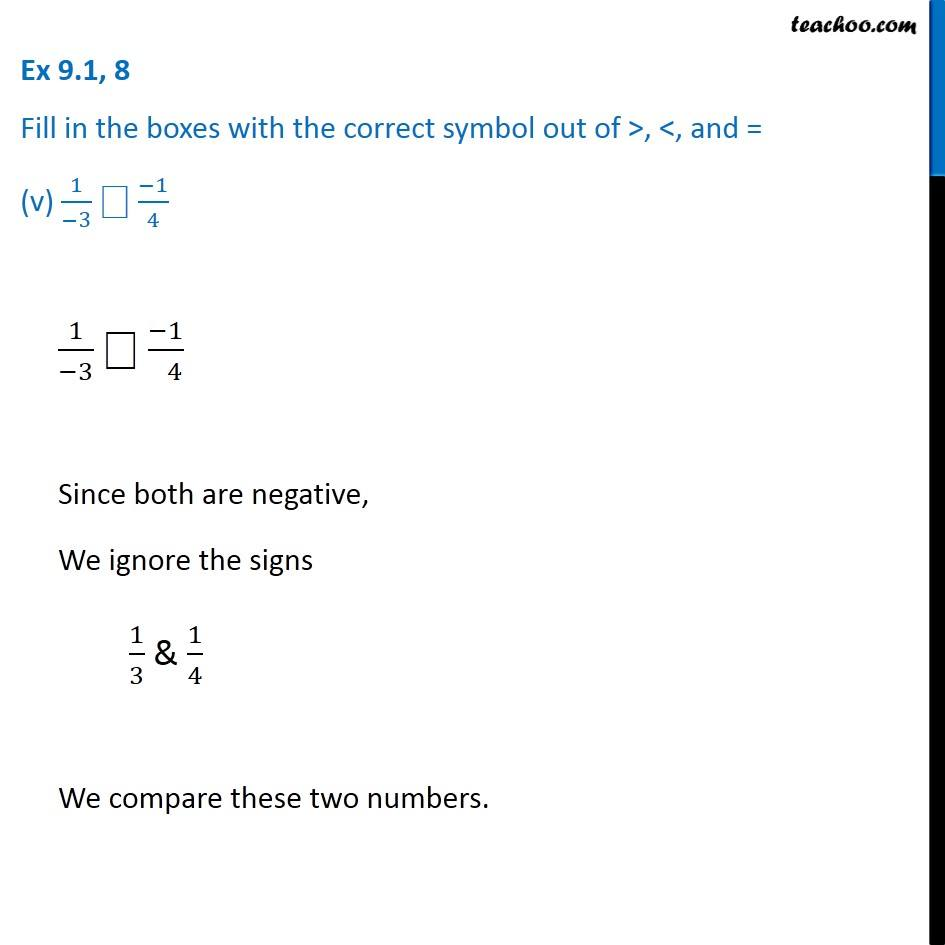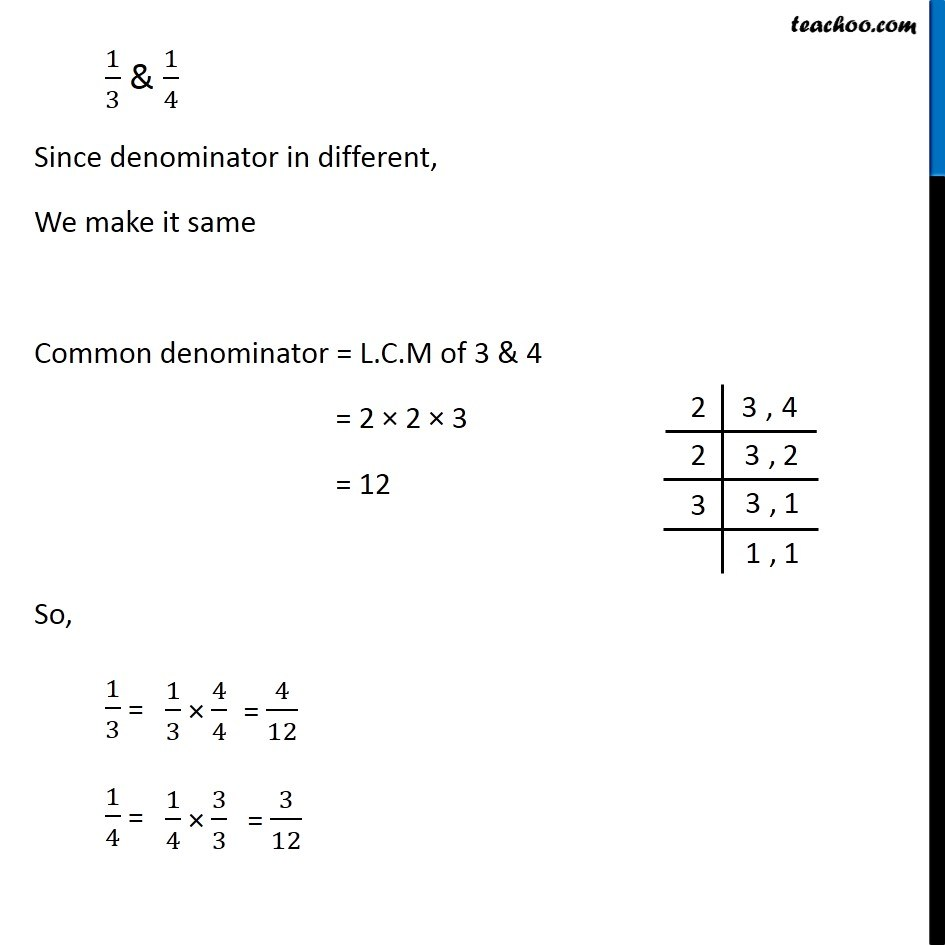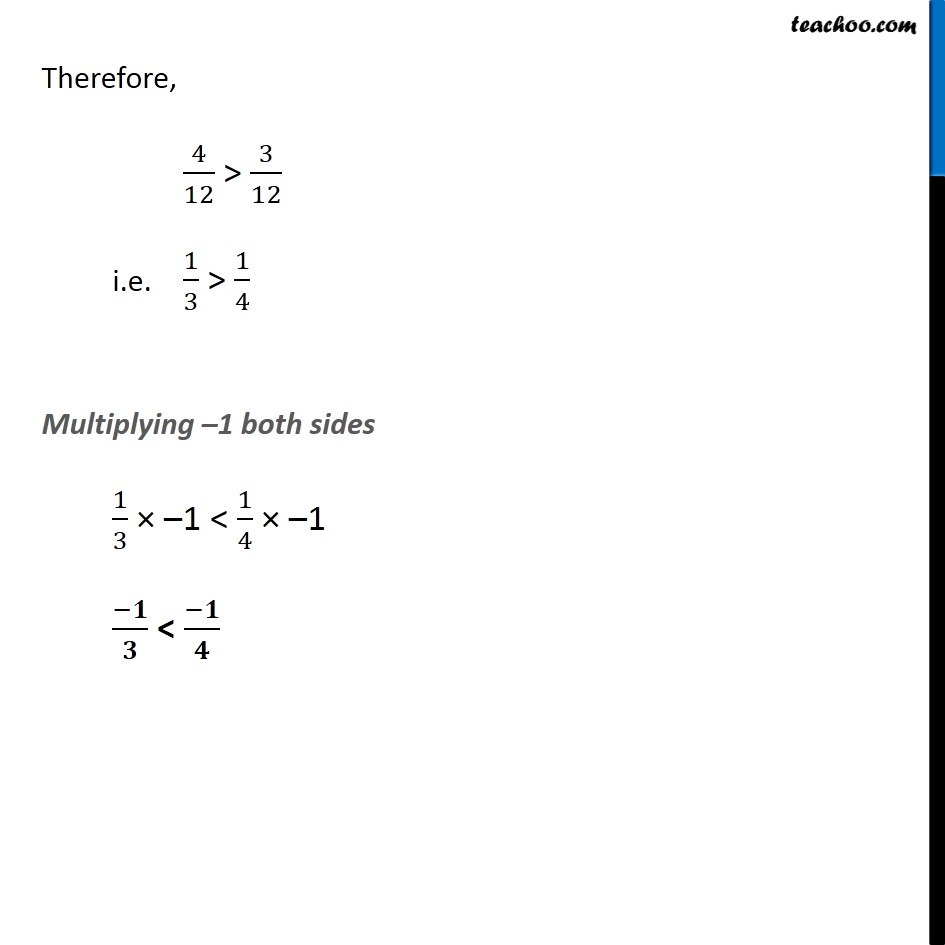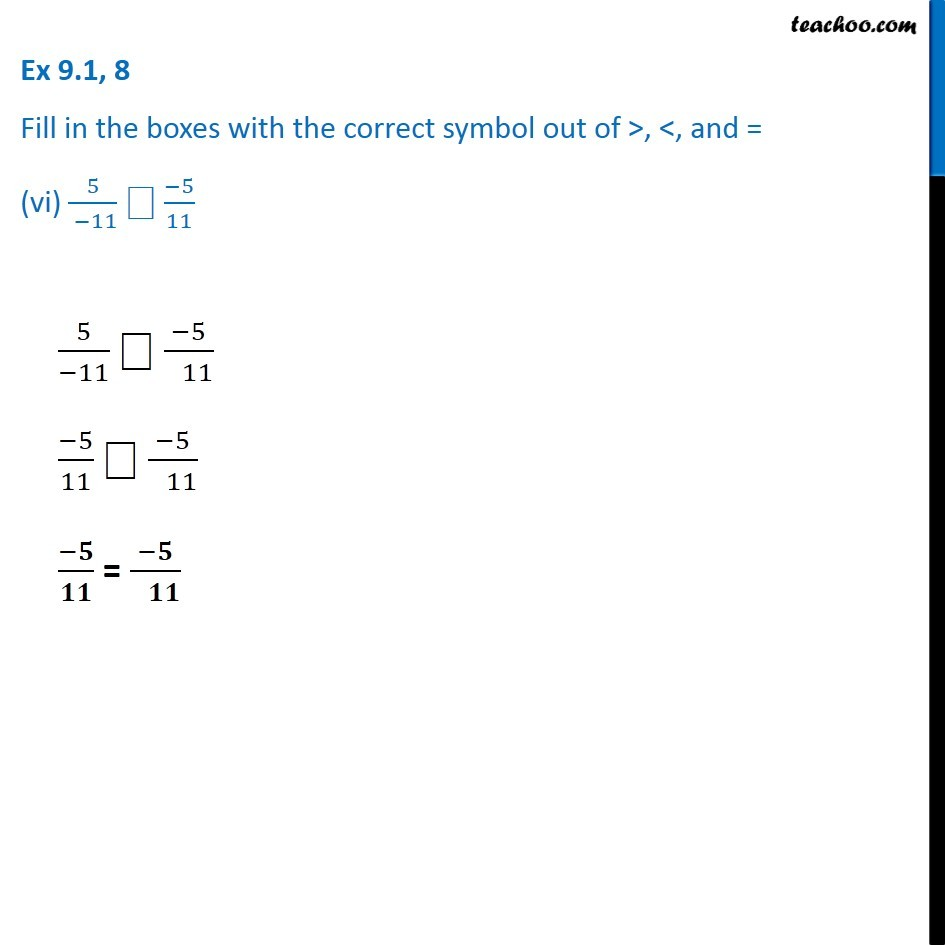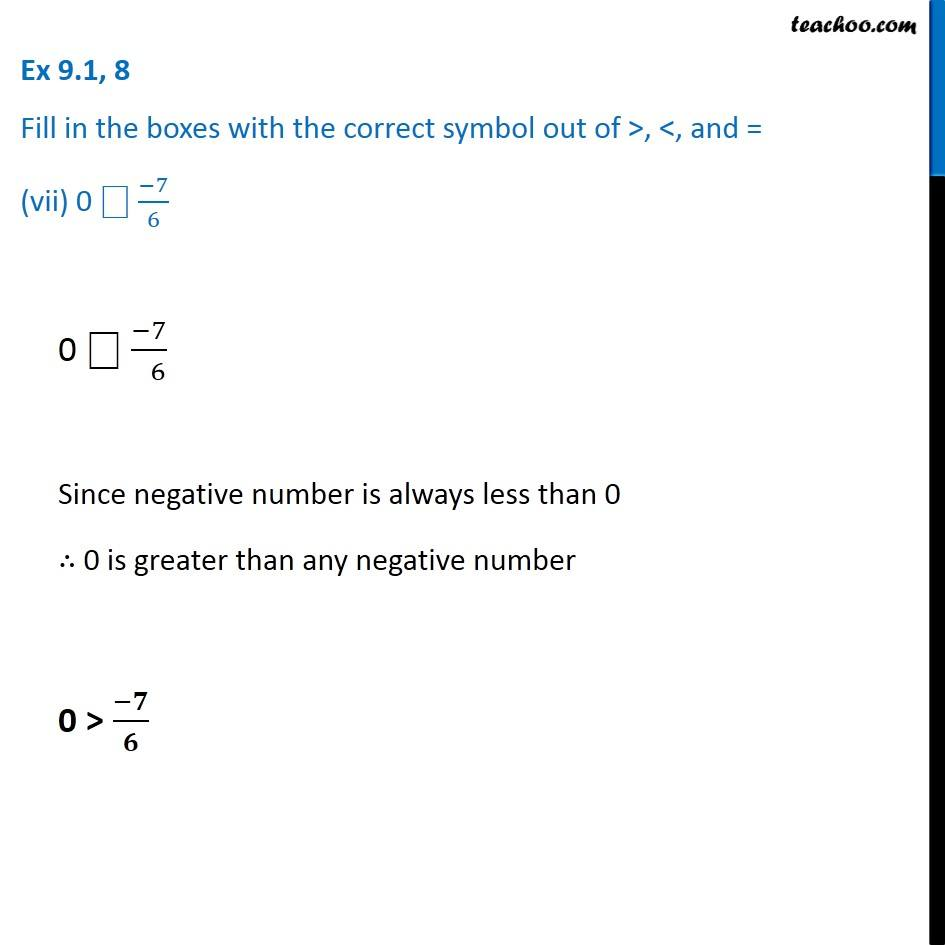Introducing your new favourite teacher - Teachoo Black, at only ₹83 per month

### Transcript

Ex 9.1, 8 - Chapter 9 Class 7 Rational Number - NCERT Solution Fill in the boxes with the correct symbol out of >, <, and = (i) (−5)/( 7) ⎕ 2/3 (−5)/7 ⎕ 2/3 Since negative number is always smaller than positive (−5)/7 < 2/3 Ex 9.1, 8 Fill in the boxes with the correct symbol out of >, <, and = (ii) (−4)/5 ⎕ (−5)/( 7) (−4)/5 ⎕ (−5)/( 7) Since both are negative, We ignore the signs 4/5 & 5/7 We compare these two numbers. 4/5 & 5/7 Since denominator in different, We make it same Common denominator = L.C.M of 5 & 7 = 5 × 7 = 35 So, 4/5 = 5/7 = Therefore, 28/35 > 25/35 i.e. 4/5 > 5/7 Multiplying –1 both sides 4/5 × –1 < 5/7 × –1 (−𝟒)/𝟓 < (−𝟓)/𝟕 Ex 9.1, 8 Fill in the boxes with the correct symbol out of >, <, and = (iii) (−7)/( 8) ⎕ 14/(−16) (−7)/8 ⎕ 14/(−16) Writing 14/(−16) in standard form 14/(−16) = (−14)/16 = (−14)/16 = (−7)/8 So, both numbers are (−7)/8 ∴ They are same (−𝟕)/𝟖 = 𝟏𝟒/(−𝟏𝟔) Ex 9.1, 8 Fill in the boxes with the correct symbol out of >, <, and = (iv) (−8)/5 ⎕ (−7)/4 (−8)/5 ⎕ (−7)/( 4) Since both are negative, We ignore the signs 8/5 & 7/4 We compare these two numbers. 8/5 & 7/4 Since denominator in different, We make it same Common denominator = L.C.M of 4 & 5 = 2 × 2 × 5 = 20 So, 8/5 = 7/4 = Therefore, 32/20 < 35/20 i.e. 8/5 < 7/4 Multiplying –1 both sides 8/5 × –1 > 7/4 × –1 (−𝟖)/𝟓 > (−𝟕)/𝟒 Ex 9.1, 8 Fill in the boxes with the correct symbol out of >, <, and = (v) 1/(−3) ⎕ (−1)/4 1/(−3) ⎕ (−1)/( 4) Since both are negative, We ignore the signs 1/3 & 1/4 We compare these two numbers. 1/3 & 1/4 Since denominator in different, We make it same Common denominator = L.C.M of 3 & 4 = 2 × 2 × 3 = 12 So, 1/3 = 1/4 = Therefore, 4/12 > 3/12 i.e. 1/3 > 1/4 Multiplying –1 both sides 1/3 × –1 < 1/4 × –1 (−𝟏)/𝟑 < (−𝟏)/𝟒 Ex 9.1, 8 Fill in the boxes with the correct symbol out of >, <, and = (vi) 5/( −11) ⎕ (−5)/11 5/(−11) ⎕ (−5)/( 11) (−5)/11 ⎕ (−5)/( 11) (−𝟓)/𝟏𝟏 = (−𝟓)/( 𝟏𝟏) Ex 9.1, 8 Fill in the boxes with the correct symbol out of >, <, and = (vii) 0 ⎕ (−7)/6 0 ⎕ (−7)/( 6) Since negative number is always less than 0 ∴ 0 is greater than any negative number 0 > (−𝟕)/𝟔